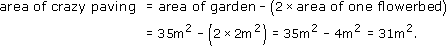Science, Maths & Technology

### Become an OU studentGeometry

Start this free course now. Just create an account and sign in. Enrol and complete the course for a free statement of participation or digital badge if available.

# 3 Areas and volumes

## 3.1 Areas of quadrilaterals and triangles

You may like to add the area formulas in this section to your notes for future reference.

The simplest areas to find are those of rectangles. The area of a rectangle is its length multiplied by its breadth. Sometimes the dimensions of a rectangle are referred to as the base and the height, instead of the length and the breadth. The area is then expressed as the base multiplied by the height.

Area of a rectangle = length × breadth = base × height

A square is a special kind of rectangle in which the length is equal to the breadth. Hence its area is the length of one side multiplied by itself, or the length of one side squared.

Area of a square = length × length = length2

For example, the area of a square mirror with sides 50 cm long is 50 cm × 50 cm = 2500 cm2.

Now consider parallelograms.

Area of a parallelogram = base × height

In the formula for the area of a parallelogram, the height is the perpendicular distance from the base to the opposite side. In order to avoid ambiguity it is sometimes called the perpendicular height rather than just the height. The height is not the length of the sloping side.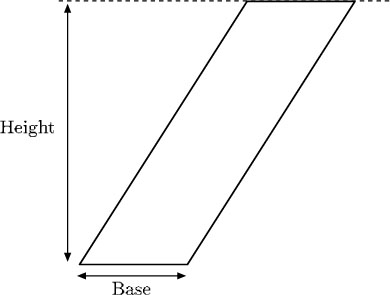At first sight, the formula for a parallelogram is quite surprising: it is the same formula as that for a rectangle. Imagine the bottom side of the parallelogram is fixed, but the top side slides along a line, as in the diagram below. The top and bottom of the parallelogram remain the same length and the same distance apart, while the other two sides lengthen or shrink. The shape always remains a parallelogram. (Notice that in one position, the parallelogram will become a rectangle – its sides will be at right angles to the base.)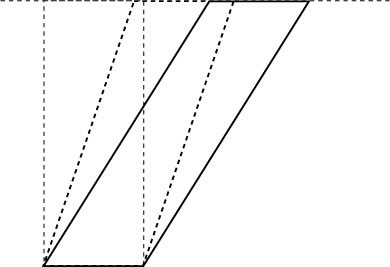The area of the parallelogram stays the same as the parallelogram shifts: it is equal to the area of the rectangle (which, of course, is given by base × height). This is easy to see by looking at the next diagram. In this, the first figure consists of two identical triangles and a parallelogram. Imagine the left-hand triangle slides to the right: it will fit above the other triangle and leave a rectangle to the left. The second figure shows the same two triangles and the rectangle. Therefore the area of the parallelogram must be the same as the area of the rectangle.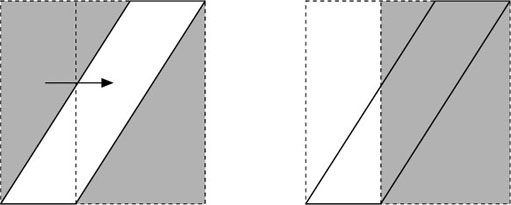Next think about the areas of triangles. Any triangle can be seen as half of a parallelogram.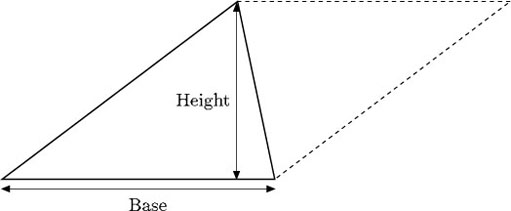So the area of a triangle is half the area of a parallelogram.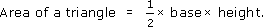Again, the height is the perpendicular height, which is now the distance from the base to the opposite corner, or vertex, of the triangle.

This formula is true for any triangle, because any triangle will be half of a parallelogram even when the perpendicular height lies outside the triangle, as below.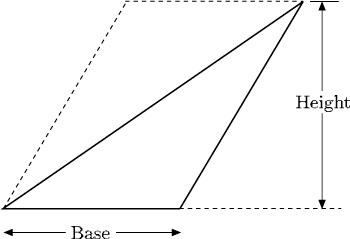If a triangle does not have a side that is horizontal, it is not clear which side is ‘the base’. The beauty of the formula for the area is that it works no matter which side is called ‘the base’. Thus the area of the following triangle can be evaluated in three ways.

You can often use what you know about the areas of rectangles and triangles to find the areas of more complex shapes.

### Example 11

The lawn shown below is trapezium-shaped. Find its area.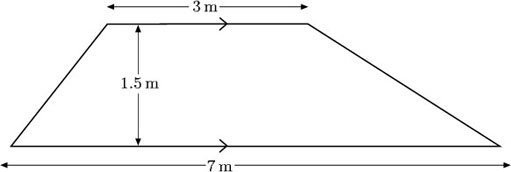Divide the lawn into three parts – a rectangle and two triangles. Then combine the two triangles into one.

So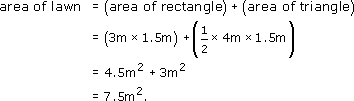### Example 12

Suppose a friend of yours decides to lay crazy paving in his garden which measures 7 m by 5 m, but he wants to leave two rectangular areas, each 2 m by 1 m, for flowerbeds. What area of crazy paving will be needed?

The first thing to do when tackling a problem like this is to draw a diagram, and to include on it all the information that has been given.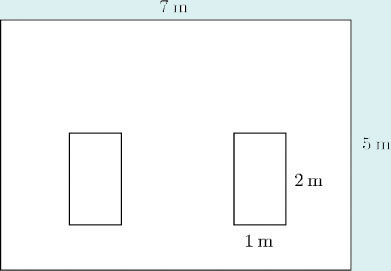Note that, as the positions of the flowerbeds have not been specified, it does not matter where they are placed.

From the diagram,

area of garden = 7 m × 5 m = 35 m2,

area of one flowerbed = 2 m × 1 m = 2 m2.

Therefore,